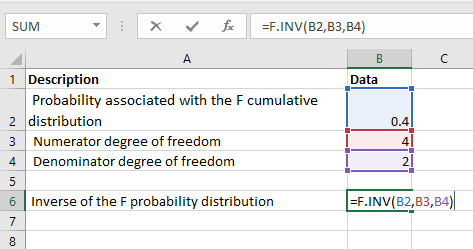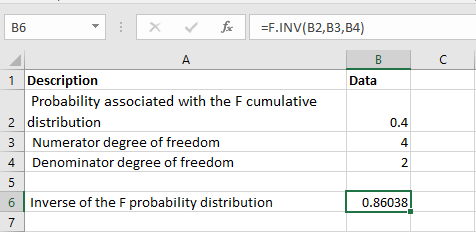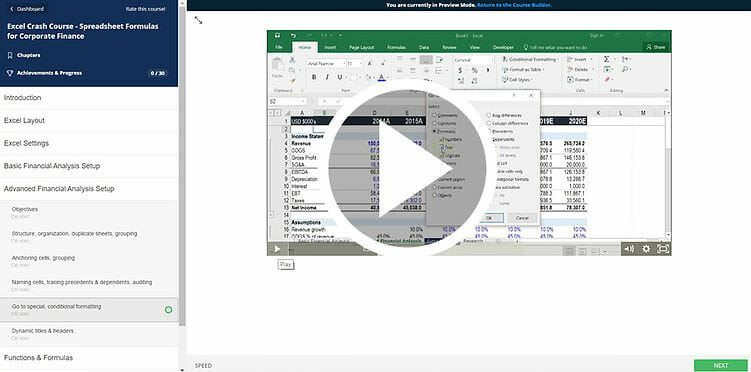# F.INV Function

Calculate the inverse of the Cumulative F Distribution for a supplied probability

## What is the F.INV (Inverse F Distribution) Function?

The F.INV function is categorized under Excel Statistical functions. It will calculate the inverse of the Cumulative F Distribution for a supplied probability.

As a financial analyst, the function is useful in understanding the variability of two data sets. One example is when we wish to analyze something such as the income diversity between two regions where a company sells its products.

### Formula

=F.INV(probability,deg_freedom1,deg_freedom2)

The F.INV function uses the following arguments:

1. Probability (required argument) – This is the probability at which we evaluate the inverse Cumulative F Distribution. The value is between 0 and 1.
2. Deg_freedom1 (required argument) – An integer specifying numerator degrees of freedom
3. Deg_freedom2 (required argument) – An integer specifying denominator degrees of freedom.

If p = F.DIST(x,…), then F.INV(p,…) = x.

Here, we need to remember that the F.INV function is the inverse of the cumulative form of the F.DIST function.

That is, if:

F.DIST(x, deg_freedom1, deg_freedom2, True ) = probability

Then, F.INV(probability, deg_freedom1, deg_freedom2) = x

### How to use the F.INV Function in Excel?

To understand the uses of the F.INV function, let’s look at an example:

#### Example

Suppose we are given the data below:

• Probability associated with the F cumulative distribution: 0.4
• Numerator degree of freedom: 4
• Denominator degree of freedom: 2

To find out the inverse of the F Probability Distribution using the F.INV function, we will use the following formula:We get the result below:### Things to remember about the F.INV Function

1. If either deg_freedom1 or deg_freedom2 is a decimal number, it is truncated to integers by Excel.
2. #NUM! error – Occurs if either:
1. The probability that is provided is less than 0 or greater than or equal to 1.
2. The argument deg_freedom1 or deg_freedom2 is less than 1.
3. #VALUE! error – Occurs when any of the arguments provided is non-numeric.
4. In MS Excel 2010, the F.INV function replaced the FINV function for better accuracy. FINV is still available in Excel but only for compatibility purposes.

### Free Excel Course

To keep learning about Excel functions and developing your skills, check out our Free Excel Crash Course!  Learn how to create more sophisticated financial analysis and modeling to become a successful financial analyst.Thanks for reading CFI’s guide to important Excel functions! By taking the time to learn and master these functions, you’ll significantly speed up your financial modeling and financial analysis. To learn more, check out these additional CFI resources:

• Excel Functions for Finance